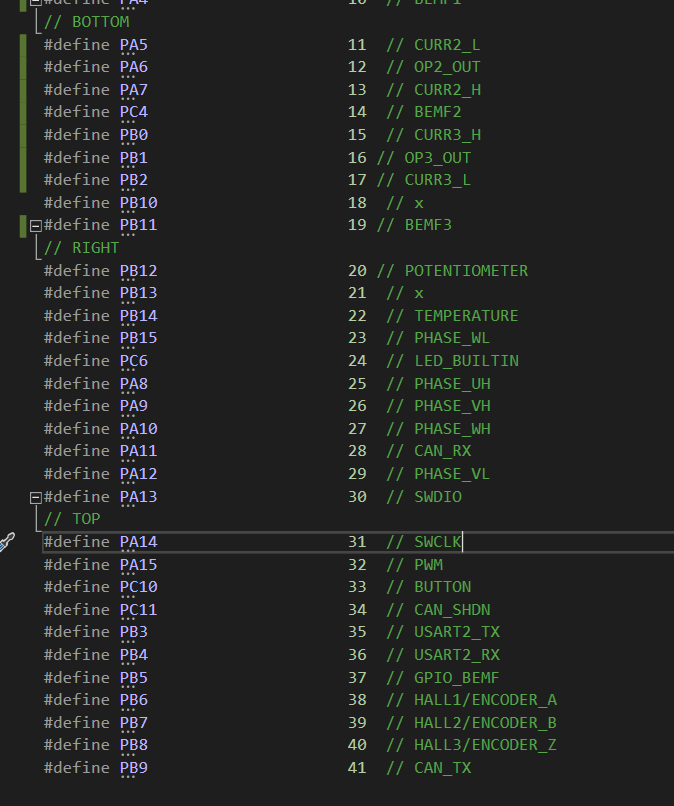# B-G431B-ESC1 IO-Pins, onboard Potentionmeter

Good Day,
I am using the B-G431B-ESC1 to power a Hub Motor with 15 Pp.
So far everything works fine. Everything works fine in open and in closed loop. Just finished PID tuning.
Now comes my question. I tried to get a analogRead of the onboard potentiometer, but no success. I thought maybe it’s a pwm-signal, so i tried to get something via the pulseIn() function, Still nothing, the output in the serial is a constant 0 for both methods. Than I thought, maybe my pin mapping is wrong, because I also tried to get an analog read of the temperature sensor.Do I have to use a function of the lib to get it working?
I need a way to control the motor externally. Therefore a analog pin or pwm pin would be great.

While I am here, can someone give me a link on how to setup the current sensoring or even better, how to setup and use the torque mode.

Don’t laugh at my code, I am a newby. xD

``````#include <SimpleFOC.h>
#include <Arduino.h>

float target = 5;
float speed = 2;
bool direction = false;
unsigned long previousMillis = 0, currentMillis = 0;

//  BLDCMotor( pole_pairs )
BLDCMotor motor = BLDCMotor(15);
//  BLDCDriver( pin_pwmA, pin_pwmB, pin_pwmC, enable (optional) )
BLDCDriver6PWM driver = BLDCDriver6PWM(A_PHASE_UH, A_PHASE_UL, A_PHASE_VH, A_PHASE_VL, A_PHASE_WH, A_PHASE_WL, true);

HallSensor sensor = HallSensor(A_HALL1, A_HALL2, A_HALL3, 15);

// Interrupt routine initialisation
void doA() { sensor.handleA(); }
void doB() { sensor.handleB(); }
void doC() { sensor.handleC(); }

void serialLoop() {
// This function is used to handle the inputs in the Serial window

while (Serial.available()) {
if (inChar == '\n') {
Serial.print("Target = "); Serial.println(target);
}
}
}

void Draw_Hall_Sensors(){
//This function is used to controll the Sensorinputs (use the Serial Plotter)
Serial.print(" ");
Serial.print(" ");
}

float Toggle_Delay(float variable, float first_value, float second_value, unsigned long interval_sec) {

currentMillis = millis();

if (currentMillis - previousMillis >= interval_sec*1000) {
previousMillis = currentMillis;
if (variable == first_value) {
variable = second_value;
}
else {
variable = first_value;
}
}
return variable;
}

void setup() {

pinMode(A_POTENTIOMETER,INPUT_ANALOG);

Serial.begin(115200);
delay(1000);

// initialize Sensor
sensor.init();
// Set Sensorinput as Interrupt
sensor.enableInterrupts(doA, doB, doC);

// power supply voltage [V]
driver.voltage_power_supply = 12;
// initialize driver
driver.init();
// link driver to the motor

// Set the voltage limit for each coil
motor.voltage_limit = 4; //[V]
// Set the velocity limit
// Set the voltage limit for sensor alignment??
motor.voltage_sensor_align = 1;

// Link the Sensor to the Motor, now closed loop

// Set the PID values for the controll loop
motor.PID_velocity.P = 1.0;
motor.PID_velocity.I = 10;
motor.PID_velocity.D = 0.0001;
motor.LPF_velocity.Tf = 0.05;

// Set control loop type to be used
motor.controller = MotionControlType::velocity;

// Set the Serial for monitoring the motor data
motor.useMonitoring(Serial);

// initialize motor
motor.init();
// initialitze the FOC algorithm
motor.initFOC(3.14, Direction::CW);

// Enable Motor and Driver
motor.enable();
driver.enable();
}

void loop() {
/*serialLoop();

motor.loopFOC();

speed = Toggle_Delay(speed, 0, 6, 5);

motor.move(speed);

Serial.print(sensor.getVelocity());
Serial.print("\t");
Serial.println(speed);
*/
Serial.print("\t");
Serial.println(pulseIn(A_POTENTIOMETER,HIGH));

}``````

Hey,

Did you get it working? TBH I can’t see what’s wrong with your example.

What happens if you simplify the program so that all you do is initialise serial output and then read the analog in for the potentiometer?
It certainly looks like the PIN number is correct in the screenshot. Where is A_POTENTIOMETER defined, and what value does it have?

pulseIn is not the right function for the potentiometer. The board also has a PWM input pin, you could attach an external PWM source to this pin, and then read that using pulseIn()

Both analogRead and pulseIn might be slow ways to get this information. Its good to get it working this way first, but you may find that calling these slow functions interferes with your motor performance. You can get around this by reading the values in more complicated “asynchronous” ways. But get it working the simple way first…

Hey,